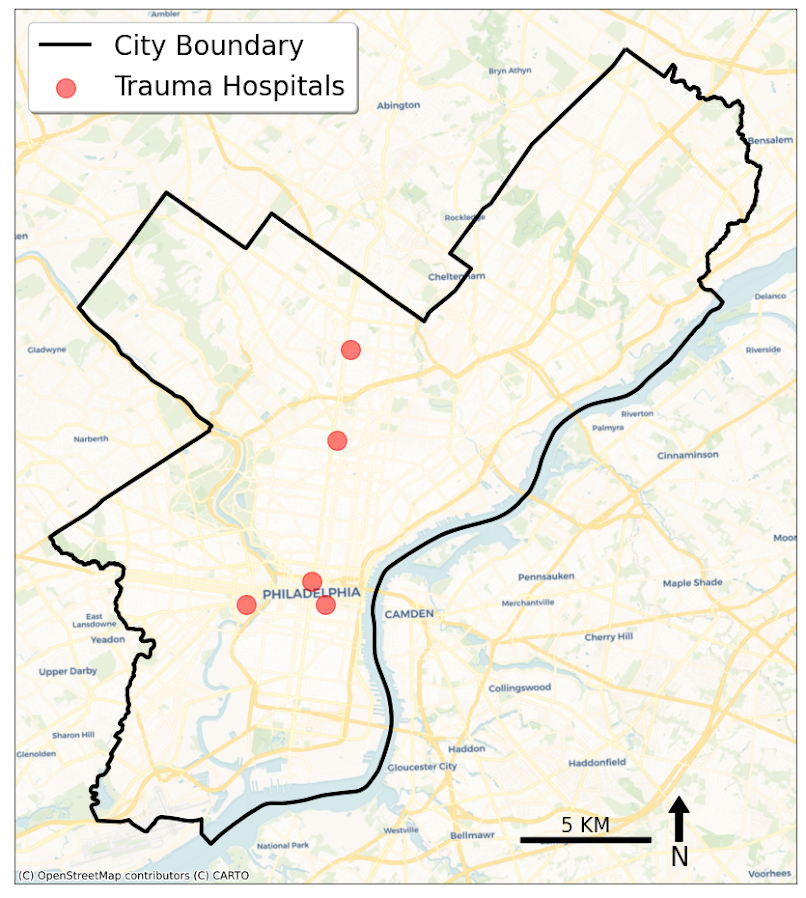# Creating a basemap in python using contextily

Me and Gio received a peer review asking for a nice basemap in Philadelphia showing the relationship between hospital locations and main arterials for our paper on shooting fatalities.

I would typically do this in ArcMap, but since I do not have access to that software anymore, I took some time to learn the contextily library in python to accomplish the same task.

Here is the map we will be producing in the end:So if you are a crime analyst working for a specific city, it may make sense to pull the original vector data for streets/highways and create your own style for your maps. That is quite a bit of work though, so for a more general solution these basemaps are really great. (And are honestly nicer than I could personally make even with the original vector data anyway).

Below I walk through the python code, but the data to replicate my paper with Gio can be found here, including the updated base map python script and shapefile data.

## Front matter

So first, I have consistently had a difficult time working with the various geo tools in python on my windows machine. Most recently the issue was older version of pyproj and epsg codes were giving me fits. So at the recommendation of the geopandas folks, I just created my own conda environment for geospatial stuff, and that has worked nicely so far.

So here I need geopandas, pyproj, & contexily as non-traditional libraries. Then I change my working directory to where I have my data, and then update my personal matplotlib defaults.

``````'''
Python script to make a basemap
'''

import geopandas
import pyproj
import contextily as cx
import matplotlib
import matplotlib.pyplot as plt
import os
os.chdir(r'D:\Dropbox\Dropbox\School_Projects\Shooting_Survival_Philly\Analysis\OriginalData'

#Plot theme
andy_theme = {'axes.grid': True,
'grid.linestyle': '--',
'legend.framealpha': 1,
'legend.facecolor': 'white',
'legend.fontsize': 14,
'legend.title_fontsize': 16,
'xtick.labelsize': 14,
'ytick.labelsize': 14,
'axes.labelsize': 16,
'axes.titlesize': 20,
'figure.dpi': 100}

matplotlib.rcParams.update(andy_theme)``````

## Data Prep with geopandas & pyproj

The next part we load in our shapefile into a geopandas data frame (just a border for Philly), then I just define the locations of hospitals (with level 1 trauma facilities) in text in the code.

Note that the background is in projected coordinates, so then I use some updated pyproj code to transform the lat/lon into the local projection I am using.

I thought at first you needed to only use typical web map projections to grab the tiles, but Dani Arribas-Bel has done a bunch of work to make this work for any projection. So I prefer to stick to projected maps when I can.

If you happened to want to stick to typical web map projections though geopandas makes it quite easy using `geo_dat.to_crs('epsg:4326')`.

``````#####################################
#DATA PREP

ph_gp = geopandas.GeoDataFrame.from_file('City_Limits_Proj.shp')

#Locations of the hospitals
hos = [('Einstein',40.036935,-75.142657),
('Hahneman',39.957284,-75.163222),
('Temple',40.005507,-75.150257),
('Jefferson',39.949121,-75.157631),
('Penn',39.949819,-75.192883)]

#Convert to local projection
transformer = pyproj.Transformer.from_crs("epsg:4326", ph_gp.crs.to_string())
hx = []
hy = []
for h, lat, lon in hos:
xp, yp = transformer.transform(lat, lon)
hx.append(xp)
hy.append(yp)
#####################################``````

## Making the basemap

Now onto the good stuff. Here I use the default plotting methods from geopandas boundary plot to create a base matplotlib plot object with the Philly border outline.

Second I turn off the tick marks.

Next I have some hacky code to do the north arrow and scale bar. The north arrow is using annotations and arrows, so this just relies on the fact that north is up in the plot. (If it isn’t, you will need to adjust this for your map.)

The scale bar is more straightforward – I just plot a rectangle on the matplotlib plot, and then put text in the middle of the bar. Since the projected units are in meters, I just draw a rectangle that is 5 kilometers longways.

Then I add in the hospital locations. Note I gave the outline a label, as well as the hospitals. This is necessary to have those objects saved into the matplotlib legend. Which I add to the plot after this, and increase the default size.

Finally I add my basemap. I do not need to do anything special here, the contextily add_basemap function figures it all out for me, given that I pass in the coordinate reference system of the basemap. (You can take out the zoom level argument at first, 12 is the default zoom for Philly.)

Then I save the file to a lower res PNG.

``````#####################################
#Now making a basemap in contextily

ax = ph_gp.boundary.plot(color='k', linewidth=3, figsize=(12,12), label='City Boundary', edgecolor='k')
#ax.set_axis_off() #I still want a black frame around the plot
ax.get_xaxis().set_ticks([])
ax.get_yaxis().set_ticks([])

x, y, arrow_length = 0.85, 0.10, 0.07
ax.annotate('N', xy=(x, y), xytext=(x, y-arrow_length),
ha='center', va='center', fontsize=20,
xycoords=ax.transAxes)

x, y, scale_len = 829000, 62500, 5000 #arrowstyle='-'
scale_rect = matplotlib.patches.Rectangle((x,y),scale_len,200,linewidth=1,edgecolor='k',facecolor='k')
plt.text(x+scale_len/2, y+400, s='5 KM', fontsize=15, horizontalalignment='center')

plt.scatter(hx, hy, s=200, c="r", alpha=0.5, label='Trauma Hospitals')

#Now making a nice legend
ax.legend(loc='upper left', prop={'size': 20})

#Now adding in the basemap imagery

#Now exporting the map to a PNG file
plt.savefig('PhillyBasemap_LowerRes.png', dpi=100) #bbox_inches='tight'
#####################################``````

And voila, you have your nice basemap.## Extra: Figuring out zoom levels

I suggest playing around with the DPI and changing the zoom levels, and changing the background tile server to see what works best given the thematic info you are superimposing on your map.

Here are some nice functions to help see the default zoom level, how many map tiles need to be downloaded when you up the default zoom level, and a list of various tile providers available. (See the contextily github page and their nice set of notebooks for some overview maps of the providers.)

``````#####################################
#Identifying how many tiles
latlon_outline = ph_gp.to_crs('epsg:4326').total_bounds
def_zoom = cx.tile._calculate_zoom(*latlon_outline)
print(f'Default Zoom level {def_zoom}')

cx.howmany(*latlon_outline, def_zoom, ll=True)
cx.howmany(*latlon_outline, def_zoom+1, ll=True)
cx.howmany(*latlon_outline, def_zoom+2, ll=True)

#Checking out some of the other providers and tiles
print( cx.providers.CartoDB.Voyager )
print( cx.providers.Stamen.TonerLite )
print( cx.providers.Stamen.keys() )
#####################################``````